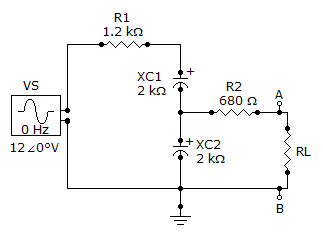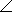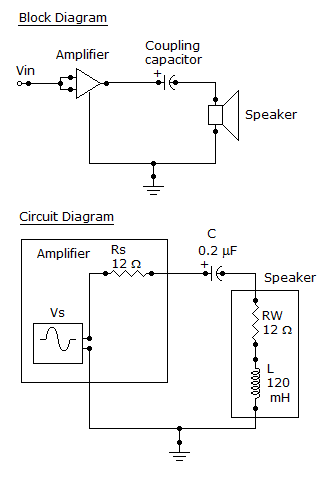# Electrical Engineering - Circuit Theorems in AC Analysis

### Exercise :: Circuit Theorems in AC Analysis - General Questions

21.

For the circuit given, determine the Thevenin voltage as seen by RL.A. 0.57416.7° V B. 5.7416.7° V C. 0.574–16.7° V D. 5.74–16.7° V

Explanation:

No answer description available for this question. Let us discuss.

22.

Determine the frequency at which the maximum power is transferred from the amplifier to the speaker in the given figure.A. 1,027 Hz B. 10,270 Hz C. 6,330 Hz D. 63,330 Hz

Explanation:

No answer description available for this question. Let us discuss.

23.

Norton's theorem gives

 A. an equivalent current source in parallel with an equivalent impedance B. an equivalent current source in series with an equivalent impedance C. an equivalent voltage source in parallel with an equivalent impedance D. an equivalent voltage source in series with an equivalent impedance